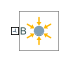# Gravitational Field

Field of force due to point mass

## Library

Forces and Torques

•## Description

This block represents the gravitational field of a point mass. This field applies a gravitational force at the center of mass of each rigid body. The force magnitude decays with the square distance from the field origin, coincident with the base port frame origin. The force on a rigid body follows from Newton’s universal gravitation law:

`${F}_{g}=-\text{\hspace{0.17em}}G\frac{Mm}{{R}_{BF}{}^{2}},$`

where:

• Fg is the force that the gravitational field exerts on a given rigid body.

• G is the universal gravitational constant, 6.67384 × 10-11 m3kg-1s-2.

• M is the total mass generating the gravitational field.

• m is the total mass of the rigid body the gravitational force acts upon.

• RBF is the distance between the source mass position and the rigid body center of mass.

The figure shows these variables. The plot shows the inverse square dependence between the gravitational force and distance.The source mass can be positive or negative. Combine multiple instances of this block to model the gravitational effects that positive and negative mass disturbances impose on a stronger gravitational field, such as a reduction in the gravitational pull of a planet due to a concentration of low-density material along a portion of its surface.

This block excludes the gravitational forces that other rigid bodies exert on the field source mass. To include these forces, you can connect Gravitational Field blocks to other rigid bodies in the model. Alternatively, you can use the Inverse Square Law Force block to model the gravitational forces between a single pair of rigid bodies.

The gravitational field is time invariant. To specify a time-varying, spatially uniform field, use the Mechanism Configuration block.

## Parameters

Mass

Total mass generating the gravitational field. The resulting gravitational forces are directly proportional to this mass. This mass adds no inertia to the model. The default value for the mass parameter is `1.0` kg.

## Ports

Frame port B represents a frame with origin at the point mass responsible for the gravitational field.

## Version History

Introduced in R2014a